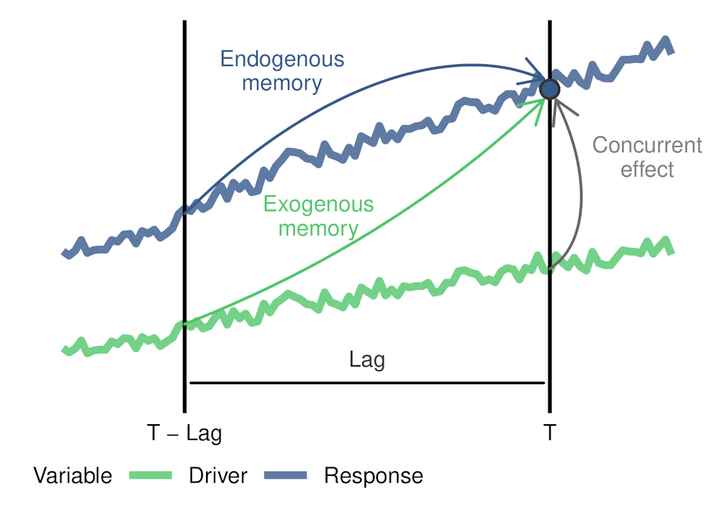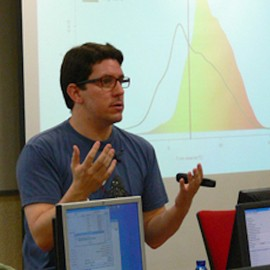# R package "memoria"Graph by Blas M. Benito

The goal of memoria is to provide the tools to quantify ecological memory in long time-series involving environmental drivers and biotic responses, including palaeoecological datasets.

Ecological memory has two main components: the endogenous component, which represents the effect of antecedent values of the response on itself, and endogenous component, which represents the effect of antecedent values of the driver or drivers on the current state of the biotic response. Additionally, the concurrent effect, which represents the synchronic effect of the environmental drivers over the response is measured. The functions in the package allow the user

The package memoria uses the fast implementation of Random Forest available in the ranger package to fit a model of the form shown in Equation 1:

Equation 1 (simplified from the one in the paper): $$p_{t} = p_{t-1} +…+ p_{t-n} + d_{t} + d_{t-1} +…+ d_{t-n}$$

Where:

• $p$ is the response variable, Pollen counts were used in this particular case..
• $d$ is an environmental Driver influencing the response variable.
• $t$ is the time of any given value of the response $p$.
• $t-1$ is the lag 1.
• $p_{t-1} +…+ p_{t-n}$ represents the endogenous component of ecological memory.
• $d_{t-1} +…+ d_{t-n}$ represents the exogenous component of ecological memory.
• $d_{t}$ represents the concurrent effect of the driver over the response.

Random Forest returns an importance score for each model term, and the functions in memoria let the user to plot the importance scores across time lags for each ecological memory components, and to compute different features of each memory component (length, strength, and dominance).The GitHub page of the package features complete examples on how to use the package. The paper published in the Ecography journal describes ecological memory concepts and the method based on Random Forest used to assess ecological memory components. The code used to generate the supplementary materials can be found in GitHub and Zenodo.

If you ever use the package, please, cite it as:

Benito, B.M., Gil‐Romera, G. and Birks, H.J.B. (2020), Ecological memory at millennial time‐scales: the importance of data constraints, species longevity and niche features. Ecography, 43: 1-10. https://doi.org/10.1111/ecog.04772